How Cheenta works to ensure student success?
Explore the Back-Story

# Test of Mathematics Solution Subjective 87 - Complex Roots of a Real Polynomial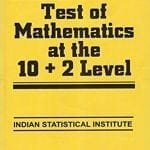This is a Test of Mathematics Solution Subjective 87 (from ISI Entrance). The book, Test of Mathematics at 10+2 Level is Published by East West Press. This problem book is indispensable for the preparation of I.S.I. B.Stat and B.Math Entrance.

Also visit: I.S.I. & C.M.I. Entrance Course of Cheenta

## Problem:

Let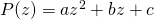, whereare complex numbers.If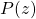is real for all real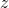, show thatare real numbers.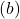In addition toabove, assume thatis not real wheneveris not real. Show that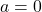.

## Solution:Asis real for all real, we have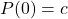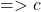is real.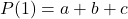is real.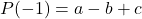is real.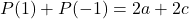is real.

As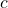is realis also real.

Similarly as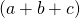is real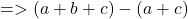is also real.

Implying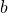is also real.

Thus allare real.Let us assume that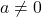.

Thus the equation can be written asLet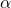be a root of the equation. Ifis imaginary that means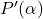is imaginary. But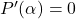, thusis real. Similarly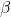, the other root of the equation, is also real.

Therefore.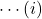Take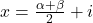Then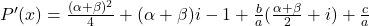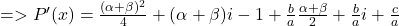Using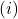, we get,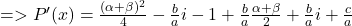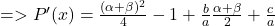Thus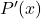is real even when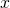is imaginary. Thus our assumption thatis wrong.

Hence Proved.This is a Test of Mathematics Solution Subjective 87 (from ISI Entrance). The book, Test of Mathematics at 10+2 Level is Published by East West Press. This problem book is indispensable for the preparation of I.S.I. B.Stat and B.Math Entrance.

Also visit: I.S.I. & C.M.I. Entrance Course of Cheenta

## Problem:

Let, whereare complex numbers.Ifis real for all real, show thatare real numbers.In addition toabove, assume thatis not real wheneveris not real. Show that.

## Solution:Asis real for all real, we haveis real.is real.is real.is real.

Asis realis also real.

Similarly asis realis also real.

Implyingis also real.

Thus allare real.Let us assume that.

Thus the equation can be written asLetbe a root of the equation. Ifis imaginary that meansis imaginary. But, thusis real. Similarly, the other root of the equation, is also real.

Therefore.TakeThenUsing, we get,Thusis real even whenis imaginary. Thus our assumption thatis wrong.

Hence Proved.

### Knowledge Partner